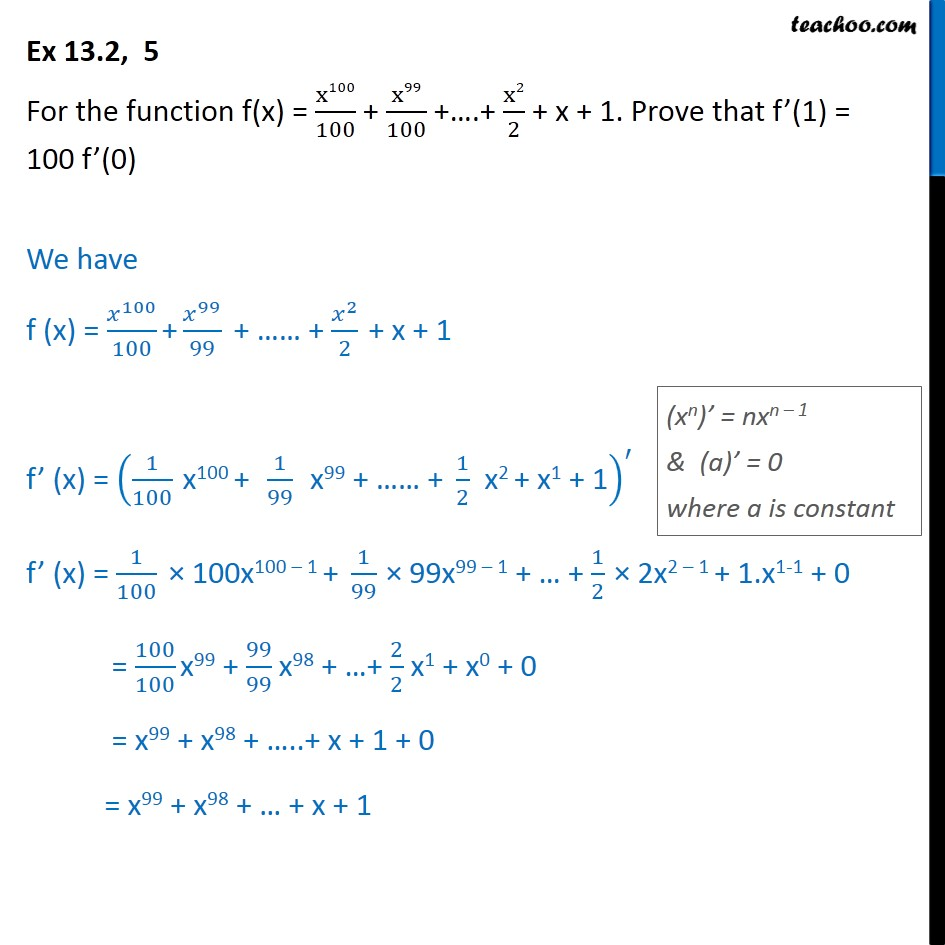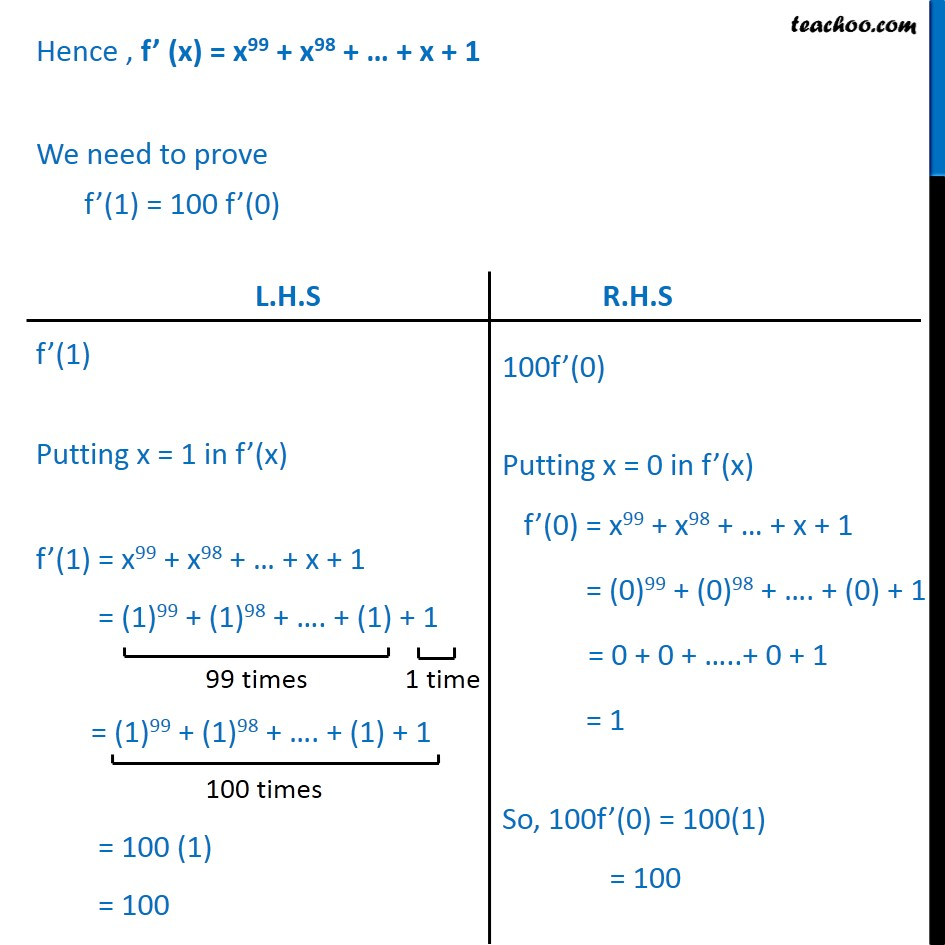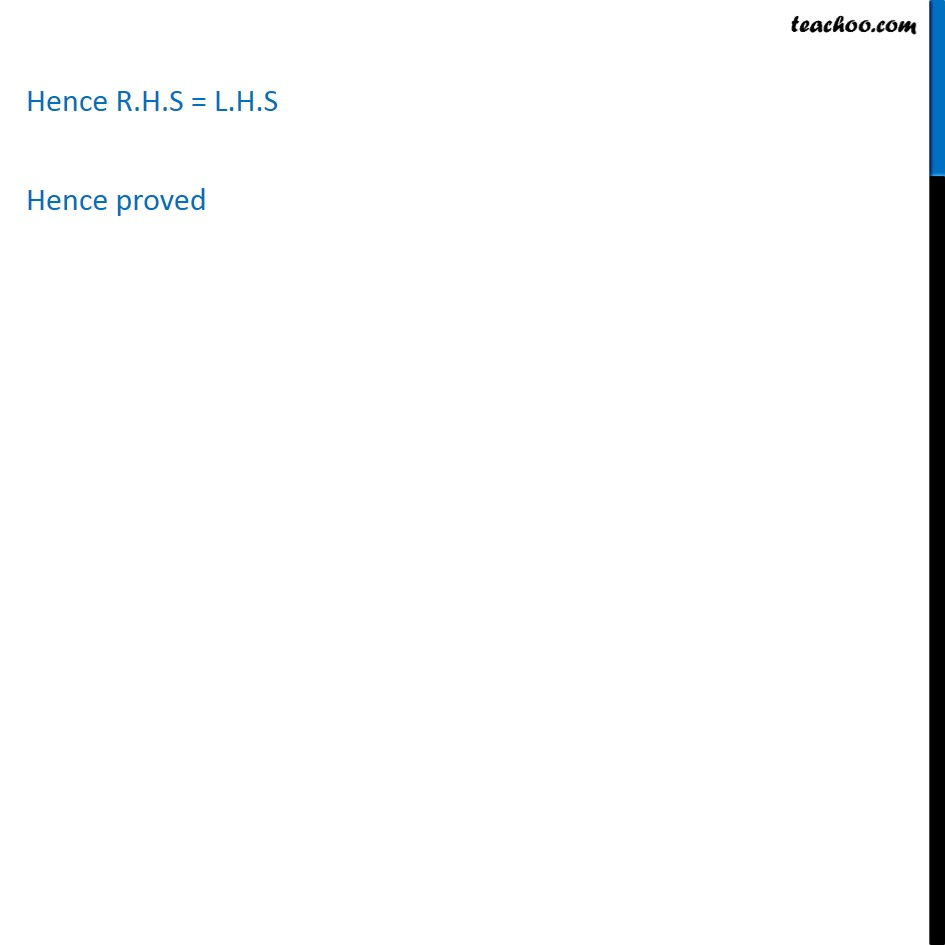1. Chapter 13 Class 11 Limits and Derivatives (Term 1 and Term 2)
2. Serial order wise
3. Ex 13.2 (Term 2)

Transcript

Ex 13.2, 5 For the function f(x) = x100﷮100﷯ + x99﷮100﷯ +….+ x2﷮2﷯ + x + 1. Prove that f’(1) = 100 f’(0) We have f (x) = 𝑥﷮100﷯﷮100﷯ + 𝑥﷮99﷯﷮99﷯ + …… + 𝑥﷮2﷯﷮2﷯ + x + 1 f’ (x) = 1﷮100﷯ x100 + 1﷮99﷯ x99 + …… + 1﷮2﷯ x2 + x1 + 1﷯﷮′﷯ f’ (x) = 1﷮100﷯ × 100x100 – 1 + 1﷮99﷯ × 99x99 – 1 + … + 1﷮2﷯ × 2x2 – 1 + 1.x1-1 + 0 = 100﷮100﷯ x99 + 99﷮99﷯ x98 + …+ 2﷮2﷯ x1 + x0 + 0 = x99 + x98 + …..+ x + 1 + 0 = x99 + x98 + … + x + 1 Hence , f’ (x) = x99 + x98 + … + x + 1 We need to prove f’(1) = 100 f’(0) Hence R.H.S = L.H.S Hence proved

Ex 13.2 (Term 2)